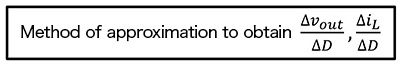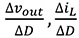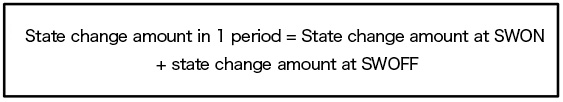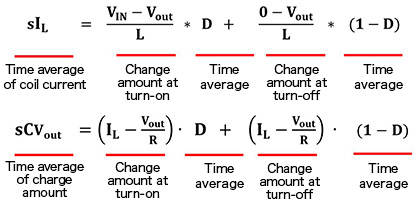# What is State-Space Averaging Method?

2017.07.06

The last time, we derived transfer functions for each block of a switching power supply. However, it was stated that, because the discussion was simplified somewhat in order to facilitate easy initial understanding, the equations obtained can be applied to the step-down mode, but not to step-up and step-up/step-down modes. This time, in order to derive transfer functions that can be applied to all the modes, we introduce the concept of “state-space averaging”.

## State-Space Averaging Method

The state-space averaging method may be slightly hard to understand. However, if this concept can be grasped, it should be possible to derive transfer functions for various modes.

To begin with, please keep in mind that the state-space averaging method isFigure 8 shows parts that are common to (shared among) and parts that differ between blocks for the step-down mode, step-up mode, and a step-up/step-down mode. As is evident, the parts that differ areNext, we explain how exactly the state-space averaging method is used. Use of the state-space averaging method assumes that “state change amounts over a single period are small”. When this assumption is valid, “the state change amounts in one period can be linearly approximated by the state change amount at SWON and the state change amount at SWOFF”, expressed as follows.In the case of a DC-DC converter, generally the coil current and the output capacitor charge are selected as state variables. A specific example for a step-down converter is shown.Here “s” is the Laplace transform; henceforth it can be regarded as s=jω. To be continued next time.